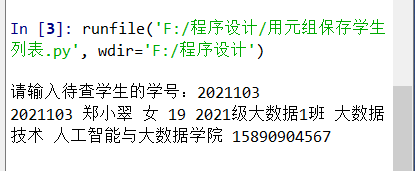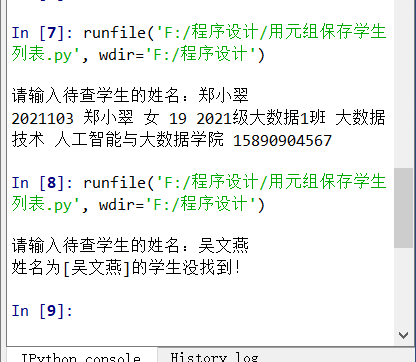• 查找学生信息（Java）
2022-03-29 20:27:38

首先创建学生类

public class Student {
private String name;
private String studentNumber;
private int age;
private String classNumber;

public Student() {

}

public Student(String name, String studentNumber, int age, String classNumber) {
this.name = name;
this.studentNumber = studentNumber;
this.age = age;
this.classNumber = classNumber;
}

public String getName() {
return name;
}

public void setName(String name) {
this.name = name;
}

public String getStudentNumber() {
return studentNumber;
}

public void setStudentNumber(String studentNumber) {
this.studentNumber = studentNumber;
}

public int getAge() {
return age;
}

public void setAge(int age) {
this.age = age;
}

public String getClassNumber() {
return classNumber;
}

public void setClassNumber(String classNumber) {
this.classNumber = classNumber;
}
}

然后

import java.util.ArrayList;
import java.util.Scanner;

public class ArrayListExample3 {
//要求实现查询学生信息的功能（通过学号）
public static void main(String[] args) {
//首先要创建学生类
ArrayList<Student> students = getInformation();
while (true) {
if (findStudent(students)) {//这个方法如果返回true说明查找成功，结束循环
break;
}
}
}

/**
* 方法用于将学生信息添加到集合中去，并打印出来
*
* @return 返回存放学生信息的集合地址
*/
public static ArrayList<Student> getInformation() {
ArrayList<Student> students = new ArrayList<>();//将所有学生信息放到集合里去
for (int i = 0; i < students.size(); i++) {//遍历集合
System.out.println(students.get(i).getName() + "\t" + students.get(i).getStudentNumber() + "\t" + students.get(i).getAge() + "\t" + students.get(i).getClassNumber());
}
return students;
}

/**
* 用于将用户输入的学号信息进行查找，来找到这个人
*
* @param students 用于传入之前存入学生信息的集合
* @return 返回的是布尔类型，看是否找到，并一直让用户查找
*/
public static boolean findStudent(ArrayList<Student> students) {
Scanner sc = new Scanner(System.in);
System.out.println("请输入你要查找的对象的学号：");
String getNumber = sc.next();
for (int i = 0; i < students.size(); i++) {
if (students.get(i).getStudentNumber().equals(getNumber)) {//这里是集合的元素的类的成员方法的比较//比较存入的学生学号是否与输入的相同
System.out.println("查找成功！！！");
printf(students.get(i));//找到了就将里面的信息打印出来
return true;
}
}
System.out.println("查找失败！！");
return false;
}

public static void printf(Student s) {//用于打印某个学生对象的信息
System.out.println(s.getName() + "\t" + s.getStudentNumber() + "\t" + s.getAge() + "\t" + s.getClassNumber());
}
}java 开发语言
更多相关内容
• java、jsp学生信息，学生成绩管理查询系统，2019最新，
• 适用初学者学习servlet/jsp框架, 了解DButils的api，页面转换，参数传递，jstl标签运用。包中含有sql脚本
• 本项目资源包括： 一、设计文档 1、功能结构 2、项目架构 3、包及Java类说明 4、数据库设计 二、功能实现 1、登陆 2、系统主界面 3、添加学生信息 4、学生信息维护 5、根据学号查找学生 6、更新学生信息 7、删除学生...
• 功能：按功能查询学生记录 作者： 日期： """ students = [] f = open('学生.txt') for line in f: line = line.replace('\n', '') student = tuple(line.split(' ')) students.append(student) id = input('...
"""
功能：按功能查询学生记录
作者：
日期：
"""
students = []

f = open('学生.txt')

for line in f:
line = line.replace('\n', '')
student = tuple(line.split(' '))
students.append(student)

id = input('请输入待查学生的学号：')
found = False
for student in students:
if (student  == id):
found = True
for i in range(len(student)):
print(student[i], end = ' ')
break
print('学号为[{}]的学生没找到！'.format(id))"""
功能：按功能查询学生记录
作者：
日期：
"""
students = []

f = open('学生.txt')

for line in f:
line = line.replace('\n', '')
student = tuple(line.split(' '))
students.append(student)

name = input('请输入待查学生的姓名：')
found = False
for student in students:
if (student == name):
found = True
for i in range(len(student)):
print(student[i], end= ' ')

print('姓名为[{}]的学生没找到！'.format(name))展开全文python 开发语言 后端
• 学生信息管理系统，实现学生信息包括：学号、姓名、性别、年龄、班级等信息。 小学生除了包括学生所有信息外，还包括英语、数学和语文成绩。 中学生除了包括小学生所有信息外，还包括地理、历史成绩、家庭住址等信息...
• 学号查询信息页面UI界面如下，当查询信息时，可在下方显示该生信息查询不到则显示“查无此人”： UI界面设计如下： package ui; import java.awt.Container; import java.awt.Font; import java.awt....

按学号查询信息页面UI界面如下，当查询到信息时，可在下方显示该生信息，查询不到则显示“查无此人”：UI界面设计如下：

package ui;

import java.awt.Container;
import java.awt.Font;
import java.awt.GridLayout;
import java.awt.event.ActionEvent;
import java.awt.event.ActionListener;

import javax.swing.JButton;
import javax.swing.JFrame;
import javax.swing.JLabel;
import javax.swing.JPanel;
import javax.swing.JTextField;

import daoImp.ClassesDaoImp;
import entity.Classes;

public class SearchByNum extends JFrame implements ActionListener {
JLabel title,number,lbl;
JTextField num;
JButton dft,cla,rtn;
JPanel line,line1,line2,line3;

public SearchByNum() {
// TODO Auto-generated constructor stub
Container con=getContentPane();
con.setLayout(new GridLayout(4,1));
line=new JPanel();
line1=new JPanel();
line2=new JPanel();
line3=new JPanel();
title=new JLabel("学生管理系统");
title.setFont(new Font("黑体", Font.PLAIN, 20));
number=new JLabel("学号：");
lbl=new JLabel();
lbl.setFont(new Font("宋体", Font.PLAIN, 15));
num=new JTextField(10);
dft=new JButton("确定");
cla=new JButton("清空");

@Override
public void actionPerformed(ActionEvent e) {
// TODO Auto-generated method stub
num.setText("");
lbl.setText("");
}
});
rtn=new JButton("返回");

@Override
public void actionPerformed(ActionEvent e) {
// TODO Auto-generated method stub
setVisible(false);
new Search().setVisible(true);
}
});
setTitle("按学号查询学生信息");
setSize(400,200);
setVisible(true);
setDefaultCloseOperation(JFrame.EXIT_ON_CLOSE);
}

@Override
public void actionPerformed(ActionEvent e) {
// TODO Auto-generated method stub
ClassesDaoImp cdi=new ClassesDaoImp();
//实例化一个Classes对象，用来接收查询结果
Classes cs=new Classes();
if(cdi.SearchByNum(num.getText())!=null) {
cs=cdi.SearchByNum(num.getText());
lbl.setText("学号："+cs.getNum()+" 姓名："+cs.getName()+" 教师："+cs.getTeacher());
}else {
lbl.setText("查无此人");
}

}

}


重写ClassesDaoImp类的按学号查询方法，实现查询功能：

package daoImp;

import java.sql.Connection;
import java.sql.PreparedStatement;
import java.sql.ResultSet;
import java.sql.SQLException;
import java.util.List;

import dao.classesDao;
import db.DBCon;
import entity.Classes;

public class ClassesDaoImp implements classesDao {
Connection cn;
PreparedStatement psm=null;
private ResultSet rs;

@Override
public boolean add(Classes classes) throws SQLException {
// TODO Auto-generated method stub
//。。。。。。

return flag;
}

@Override
public boolean delete(String num) {
// TODO Auto-generated method stub
//。。。。。。
return flag;
}

@Override
public boolean update(Classes classes) {
// TODO Auto-generated method stub
//。。。。。。
return flag;
}

@Override
public Classes SearchByNum(String num) {
// TODO Auto-generated method stub
DBCon dbcon=new DBCon();
cn=dbcon.getConnection();
Classes cs=new Classes();
String sql="select * from classes where num=?";
try {
psm=cn.prepareStatement(sql);
psm.setString(1, num);
rs=psm.executeQuery();
if(rs.next()) {
//将rs返回的的值值赋给cs对象
cs.setNum(rs.getString(2));	//2表示索引值，是rs指针指向的行的第二个值
cs.setName(rs.getString("name"));//利用列名读取数据
cs.setTeacher(rs.getString(4));
return cs;
}
psm.close();
cn.close();
} catch (SQLException e) {
// TODO Auto-generated catch block
e.printStackTrace();
}

return null;
}

@Override
public List<Classes> sreachByTeacher(String teacher) {
// TODO Auto-generated method stub
return null;
}

}


PS:
1.执行产生单个结果集的sql语句（如SELECT语句）使用executeQuery()方法
2.executeQuery()返回ResultSet对象，且ResultSet对象一次只读取一个数据行，需要使用next()方法指向下一行再继续读取操作。对于每一行数据，可以使用索引或列名获取字段值

展开全文java
• 本文实例为大家分享了python mysql学生信息查询系统的具体代码，供大家参考，具体内容如下import pymysql#import redis#pool = redis.ConnectionPool(host='IP地址', port=6379, decode_responses=True)while True:...

本文实例为大家分享了python mysql学生信息查询系统的具体代码，供大家参考，具体内容如下

import pymysql

#import redis

#pool = redis.ConnectionPool(host='IP地址', port=6379, decode_responses=True)

while True:

while True:

# print('=' * 15)

user = str(input('请输入用户名：'))

passwd = str(input('请输入密码：'))

# print('=' * 15)

if passwd == 'root':

print('=' * 20, '\n学生查询系统\n'

'1.学院类型信息\n')

print('=' * 20)

break

else :

print('--请重新输入密码--')

num = int(input('请输入功能相对应的数字：'))

print()

db = pymysql.connect('IP地址','MySQL用户名','MySQL密码','数据库', charset='utf8')

cursor = db.cursor()

if num == 1:

sql = 'select type_id,name from school_type'

cursor.execute(sql)

results = cursor.fetchall()

print('学院序号 学院名称')

for row in results:

print(row)

print()

num1 = int(input('请输入你想查看的学院对应数字：'))

while True:

if num1 == 1:

print('-' * 15,'\n1 查看该学院介绍\n'

'2 查看该学院开设专业\n'

'0 返回上一层')

print('-' * 15)

num2 = int(input('请输入对应功能的数字：'))

if num2 == 1:

sql1 = 'select name,introduction from school_type'

cursor.execute(sql1)

results1 = cursor.fetchall()

print('学院名字 学院简介')

for row1 in results1:

print(row1)

elif num2 == 2:

sql2 = 'select * from eie'

cursor.execute(sql2)

results2 =cursor.fetchall()

print('序号 名字 班级数量')

for row2 in results2:

print(row2)

break

num3 = int(input('请输入想要查询专业名前所对应的数字：'))

if num3 == 1:

sql3 = 'select class_id,classname,number,manager from class join class_manager on(class.class_id = class_manager_id)'

cursor.execute(sql3)

results3 = cursor.fetchall()

print(' 名称 人数 班主任')

for row3 in results3:

print(row3)

num4 = int(input('请输入想要查询班级前所对应的数字：'))

while True:

if num4 == 1:

print('-' * 20,'\n1、查看班级所有人信息\n'

'2、添加学生信息\n'

'3、删除学生信息\n'

'4、修改学生信息\n'

'0、退出系统')

print('-' * 20)

num5 = int(input('请选择功能(序号)：'))

if num5 == 1:

sql4 = 'select student_name,sex,student_num,evaluation from cloud_one'

cursor.execute(sql4)

results4 = cursor.fetchall()

print('姓名 性别 学号 评分')

for row4 in results4:

print(row4)

elif num5 == 2:

print('您选择了添加学生信息功能')

name = str(input('请输入学生姓名：'))

sex = str(input('请输入学生性别：'))

studentnum = int(input('请输入学生学号(学号不可重复)：'))

pf = int(input('请输入学生评测分数：'))

sql5 = 'insert into cloud_one(student_name,sex,student_num,evaluation) values("%s","%s",%d,%d)' % (name,sex,studentnum,pf)

print(sql5)

try:

cursor.execute(sql5)

db.commit()

except:

db.rollback()

print('******已添加成功******')

elif num5 == 3:

print('您选择了删除学生信息功能')

num6 = int(input('请您输入要删除的学号：'))

sql6 = 'delete from cloud_one where student_num = %d'%num6

try:

cursor.execute(sql6)

db.commit()

except:

db.rollback()

print('******已删除成功******')

elif num5 == 4:

print('您选择了修改学生信息功能')

num7 = int(input('请您输入要修改的学生学号'))

sql7 = 'select student_name,sex,student_num,evaluation from cloud_one where student_num=%d'%num7

cursor.execute(sql7)

results5 = cursor.fetchall()

print(' 姓名 性别 学号 评分')

print(results5)

xm = str(input('请输入您要更改的姓名：'))

xb = str(input('请输入您要更改的性别：'))

xh = int(input('请输入您要更改的学号：'))

pf = int(input('请输入您要更改的评分：'))

sql8 = 'update cloud_one set student_name="%s",sex="%s,student_num=%d,evaluation=%d' % (xm,xb,xh,pf)

print(sql8)

try:

cursor.execute(sql8)

db.commit()

except:

db.rollback()

print('*****已更改成功****')

elif num5 == 0:

num8 = str(input('******你真的要离开宝宝吗******(Y/N)：'))

if num8 == 'Y':

break

else:

continue

查看信息的时候，上面程序可以用pandas中的一种数据结构DataFrame优化一下。

可以网上了解一下DataFrame！！！！

这是我本人做的一些与上面程序有关的数据库。因为初学者，仅供参考以上就是本文的全部内容，希望对大家的学习有所帮助，也希望大家多多支持。

展开全文• 在之前的博客中我写过关于简易的查询四级成绩的web程序，在这里，我们连接了数据库，写一个通过输入学生的学号查询学生成绩的web小程序。...四级是实现Servlet接口，学生信息查询是继承HttpServlet...jsp servlet web
• } } if(c==false) cout该学生不存在"<>b; for(i=1;i>b;for(i=1;i>b; for(i=1;i>ch; s=0; while(s==0) { switch(ch) { case'a': add(l); break; case'b': delete(l); break; case'c': modify(l); break; case'd': ...
• 一个关于学生信息管理系统~~可实现查询，修改，删除等等！输入学生学号可以查询学生的学号，姓名，年龄，性别等等，很好的一个C++编写的学生信息管理系统
• 是用java图形界面做的 实现了学生信息的添加 删除 修改（根据学号或姓名）查询等功能，用的是mySQL数据库
• 学生信息管理系统 C 语言编程【问题描述】学生信息的管理是每个学校必须具有的管理功能，主要是对学生的基本情况及学习成绩等方 面的管理。该系统模拟一个简单的学生管理系统，要求对文件中所存储的学生数据进行各种...
• Stringname=request.getParameter("name");...ResultSetrs=stmt.executeQuery("select*fromstudentwhere学生学号='name'"以上代码中的ResultSetrs=stmt.executeQuery("s...String name=request.getParameter("nam...
• 定义一个包含学生信息学号，姓名，成绩）的顺序表和链表（二选一），使其具有如下功能：(1) 根据指定学生个数，逐个输入学生信息；(2) 逐个显示学生表中所有学生的相关信息；(3) 根据姓名进行查找，返回此学生的...
• 定义一个包含学生信息学号，姓名，成绩）的顺序表，使其具有如下功能： (1) 逐个输入学生信息，建立信息表； (2) 显示信息表中所有学生的相关信息； (3) 根据姓名进行查找，返回此学生的学号和成绩； (4) 给定一个...
• 2.多表查询 3.php变量在sql的引用 二.详解: 1.插入数据时由于涉及多次操作考虑自写insert()函数,提高效率 function insert($table,$arr,$link) {$keys=implode(',',array_keys(\$arr));//根据逗号分割数组的...sql 数据库
• 学生信息包括：学号，姓名，年龄，性别，出生年月，地址，电话，E-mail 等。 试设计一个学生信息管理系统，使之能提供以下功能...4、学生信息查询功能 按学号查询 按姓名查询 5、学生信息的删除与修改 6、运用冒泡排序
• 1）查询学生信息  按学号或姓名查询 2）添加学生信息 3）修改学生信息 4）删除学生信息 5）对学生按照学号排序并输出其信息 #include<stdio.h> #include<stdlib.h> #include<string.h> #define ...数据结构 链表 c语言
• 设计一个GUI界面的学生信息管理系统，方便保存学生的部分信息。 有五类功能： 1、 增加学生信息：输入姓名、学号、年龄、手机号 ...4、 查询学生信息：以学号为索引 5、 显示学生信息：一条一条整页显示。
• 主界面输入2时，进入查询学生信息模块，根据学生学号，对学生信息进行查询。 在主界面输入3时，进入到删除学生信息模块，输入需要删除的学生学号，即可在文件中将该学号的所有信息删除。 当在主界面输入4时，系统...
• 请编写一个程序，使用字典存储学生信息学生信息包含学号和姓名，请根据学生学号从小到大输出学生信息 目录 列表输出 这一题就可以借助于字典的推导式 通过字典输出的另一种方法 第一种方法 列表输出 dic...python list
• 进入系统，实现个人成绩的查询， 对个人基本信息的增删改 学生表　学号　密码　姓名　班级　电话　住址 成绩　学号　课程　成绩 管理员　账号　密码 数据库名：xs xuesheng xuehao password xingming　banji ...
• //输入学号 for(int i=0;i;i++) { base=0; top=n-1; while(base) { mid=(base+top)/2; if(b[i]>stu[mid].no) base=mid+1; else if(b[i][mid].no) top=mid-1; else if(b[i]==stu[mid].no) {flag=mid;...
• python查找学生对应学号_python+mysql实现学生信息查询系统 import pymysql #import redis #pool = redis.ConnectionPool(host=‘IP地址’, port=6379, decode_responses=True) while True: while True: print(’=’...python
• 这是一个学生信息管理系统，学生信息主要包括：学号，姓名，性别，出生日期，班级；暂时就只有这些信息，若后续需要，可以相应的进行添加。 该项目主要采用的技术是 Java GUI 设计，这个地方主要用 Java 工具 ...java mysql jdbc
• 模拟使用map进行学生信息学号、姓名、性别、年龄、专业）存储，key值是学生的学号，value值是对应学生的信息，要求此map中保存的数据根据学号自动升序排序。 package com.zut.Nove; import java.util.*; public ...java
• 以下内容是本人将近一个星期的劳动成果C语言程序设计作为本学期最后一次实训 设计内容学生信息查询系统 可实现 1 录入学生信息 2 显示学生成绩 3 修改学生成绩 4 删除学生成绩 5 按学号查询学生信息 6 按学好显示......